# Sieve method

(diff) ← Older revision | Latest revision (diff) | Newer revision → (diff)
Jump to: navigation, search

A general method in number theory which generalizes the principle of sifting compound numbers from the natural series (see Eratosthenes, sieve of). The problem of the sieve method consists in evaluating for a finite setof integers the quantity of those elements that are not divisible by any prime numberfrom some setof prime numbers. The "sifting" function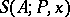, which denotes the number of such elements fromunder the additional condition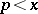, is often estimated by using information about the number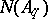of elements of a set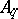. This setconsists of the elements ofthat are divisible by a square-free number. When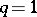,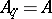. Therefore the more general sifting functionis usually estimated.

The choice of an expected value forin the form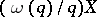, whereis the expected value forand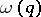is a multiplicative function, is governed by the fact that the degree of errorshould be relatively low. If, moreover,(at least "on the average" ), thenis called the dimension of the sieve.

The most advanced branch of the general theory of the sieve method and its applications is that of the linear sieve (when). There are various specializations, the most important of which are the Brun sieve and the Selberg sieve.

When the sieve method is applied to additive problems (see Additive number theory), the sifting function must be estimated from below as well as from above. Estimates from below may be based on the logical combinatorial identity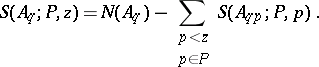The most precise estimates from below are obtained by invoking combinatorial considerations associated with the use of weight functions. A strong result in the applications of the sieve method with weight functions is that each sufficiently large even numberis representable in the form, whereis a prime number and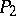contains at most two prime factors.

How to Cite This Entry:
Sieve method. Encyclopedia of Mathematics. URL: http://encyclopediaofmath.org/index.php?title=Sieve_method&oldid=12559
This article was adapted from an original article by B.M. Bredikhin (originator), which appeared in Encyclopedia of Mathematics - ISBN 1402006098. See original article